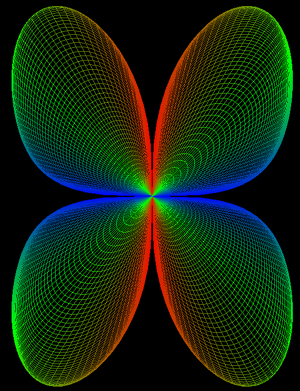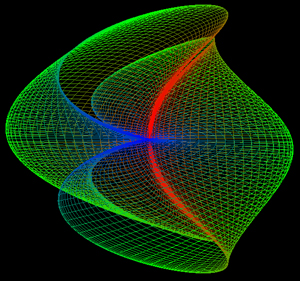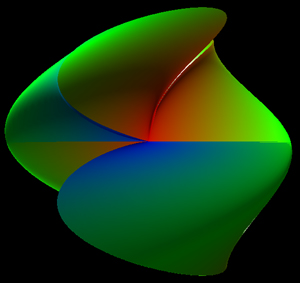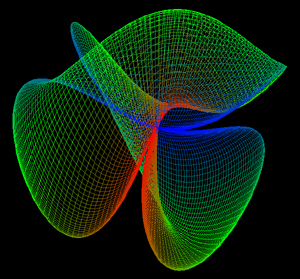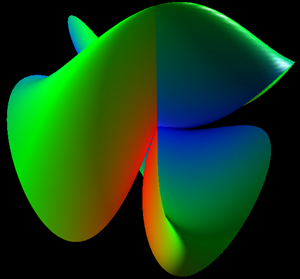# Implicit Minimal Lemnescate

By Roger Bagula
Graphics by Paul Bourke
August 2002
x = cos(v) sqrt(|sin(2 u)|) cos(u)
y = cos(v) sqrt(|sin(2 u)|) sin(u)
z = x2 - y2 + 2 x y tan2(v)
0 <= u <= pi, 0 <= v <= pi